MATHEMATICS / PRIMARY

# Counting with fingers: An introduction to number systems

9 NOVEMBER 2021

Chapter 1: How do we count and represent numbers?

In modern times, we use Arabic numerals to represent numbers. There are ten symbols that are used to represent numbers: 0, 1, 2, 3, 4, 5, 6, 7, 8, and 9. These ten symbols are sufficient to represent any number in writing. Each number is represented by a unique combination of symbols, and each combination of symbols represents a unique number.

How is this possible? Let us start by examining how numbers are represented in our Arabic number system.

The Arabic number system is known as a base-10 number system, because there are ten different digits used. The value of a digit is equal to the base multiplied by the value of its place. Take the number 314 for example: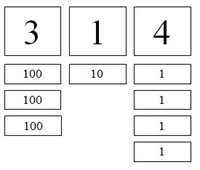Although 4 is bigger than 3, in the number 314, the number 3 has a greater value than the number 4. Of course, 3 is in the hundreds place, 1 is in the tens place, and 4 is in the ones place.

Each digit is multiplied by its place value to give its value in the number. As 3 is in the hundreds place, its value is: 3 × 100 = 300. Likewise, for the rest of the number, we can see that 314 = 3 × 100 + 1 × 10 + 4 = 300 + 10 + 4.

What determines the value of each place? Using our ten digits, why can’t the place values be 1, 10, 20; or 1, 100, 10000?

Earlier, we mentioned that our base-10 number system allows us to represent each number with a unique combination of symbols. If the places were 20, 10, and 1, then I could represent the number 61 in two different ways:

“301” = (3 × 20) + (0 × 10) + 1 = 60 + 1 = 61

“221” = (2 × 20) + (2 × 10) + 1 = 40 + 20 + 1 = 61

Since I can use represent numbers using two different ways, this is not a valid base system. If the places were 10000, 100, and 1, the following problem would result: I cannot represent the number 61 using this number system.

(A × 10000) + (B × 100) + (C × 1) = 61

A, B, and C must be digits of a three-digit number. There are only 10 digits in our base-10 number system, from 0 to 9. If A or B are 1 or higher, the result would be greater than 61. C can at most be 9. Hence, 61 cannot be represented in this number system, and this would not be valid either.

Because we have ten symbols, each place in our number system is worth ten times as much as the place to the right of it. Therefore, in base-10, we have a ones place, tens place, hundreds place, and so on.

Chapter 2: Other ways of representing numbers

Computers are unable to process base-10 numbers, as they operate based on electricity. They use a base-2 number system instead, with two different digits: 0 and 1. 1 represents the presence of electrical flow whereas 0 represents the absence of electrical flow. As electrical flow can be started and stopped billions of times each second, the computer can calculate numbers very fast.

Earlier, we mentioned that in a base-10 number system, each place is worth ten times as much as the place to the right of it. In a base-2 number system, each place is worth two times as much as the place to the right of it. Thus, in base-2, we have a ones place, twos place, fours place, eights place, and so on.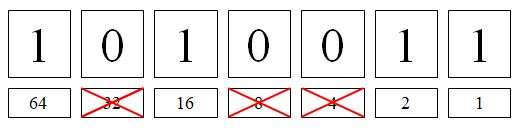1010011 in base-2 = 1 + (1×2) + (0×4) + (0×8) + (1×16) + (0×32) + (1×64) = 83 in base-10.

Mathematicians represent the base of a number by adding the base in subscript at the end of the number. We can thus represent the above information as:

10100112­ ­ = 8310.

If no subscript is added at the end of the number, the number is base-10 by default. Hence 8310 can just be written as 83.

Remember that in base-2, there are only two digits, 0 and 1. Thus, it is meaningless to write 832, as neither 3 nor 8 are digits in base-2.

Chapter 3: Decimals in other bases

In base-10, we represent decimals by adding the decimal digits after a decimal point. Each digit still has a value of ten times the digit to the right of it. Thus, the tenths place is to the right of the ones place, the hundredths place is to the right of the tenths place, and so on.

How do we represent fractions such as 1/4 in decimal form? We can convert 1/4  into 25/100 , which is equal to 2/10 + 5/100. Therefore,  is equivalent to 0.25, as shown below.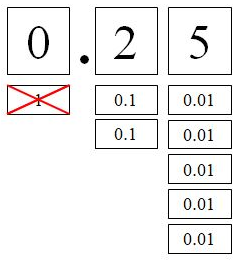1/4 = (2×0.1) + (5×0.01) = 0.25

While most countries around the world today use base-10, some early human civilisations used other bases in their number systems. In Nepal and Nigeria, people used a base-12 number system, while in the Babylonian civilisation, people used a base-60 number system. In fact, linguists and historians have theorised that English used a base-12 number system in the past, as the words for “eleven” and “twelve” do not follow the pattern of words ending in    “-teen”.

Each base has its advantages and disadvantages. In base-10, very few numbers can be represented exactly as decimals. For example,  cannot be represented as a decimal in base 10 exactly, because 1/3  = 0.333 333… which repeats forever.

How is 1/3  represented in base-12? In base-12, we need to have twelve symbols for the twelve digits. Similar to base 10, the digits 0-9 will represent the numbers from 0 to 9. We can use A to represent a quantity of 10, and B to represent a quantity of 11. Thus,

BA12 = (11×12) + (10×1) = 14210.

For decimals, the place to the right of the decimal point represents the twelfths place, as its value is one-twelfths of the ones place. Further to the right is the hundred-forty-fourth place, which is one-twelfths of the twelfths-place. Thus, 0.112 = 1/12  in base-10, and 0.0112 = 1/144  in base-10.

A quantity of 1/3 is simply written as 0.4 in base-12, as 1/3 = 4/12 :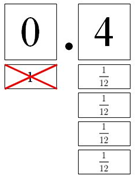Using a bigger base allows us to express more fractions as simple decimals. However, it also requires more symbols. In base-60, 60 different symbols are used. These are shown below: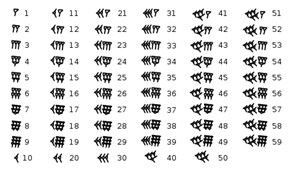The Babylonians did not have a symbol to represent zero. In modern times, some mathematicians writing Babylon numerals simply use the Arabic 0 in its place.

Numbers bigger than 60 were separated by a space, thus, the number 31415 could be represented by: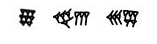In base-60, the place to the left of the ones place has a place value of 60, and further to the left is the 3600-place, as 3600 equals 60 × 60. Thus, 31415 = (8×3600) + (43×60) + (35×1).

Chapter 4: Arithmetic in different bases

Let us conclude by seeing how simple mathematical operations are done in different bases. In modern times, it is easy to evaluate a sum of 7312 + 6612, as we just have to convert the numbers to base-10: 7312 = 87 and 6612 = 78, thus 87 + 78 = 165 = 11912. However, early people had no knowledge of base-10, and thus were unable to make such a conversion. All calculations thus had to be done in base-12.

Addition in base-12 is done similarly as base 10: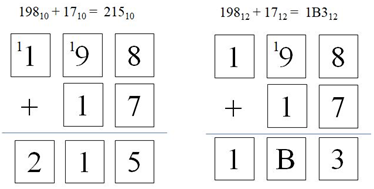Remember that in base-12, there are twelve symbols: the digits 0-9 represent the quantities 0 through 9 just like in base-10, while A is used for 10 and B is used for 11.

To add two numbers in base-12, we start from the rightmost place (which is the ones place), similar to base 10. In base-12, 812 + 712 = 1312. The 3 is written down, while 1 is carried over to the next column, the twelves place. In the twelves place, 112 + 912 + 112 = B12, without any carry-over to the next column. To the right of the twelves place is the hundred-forty-fours place, as 144 = 12 × 12.

Thus, the complete sum is 19812 + 1712 = 1B312, and the answer of 1B312 represents       (1×144) + (11×12) + (3×1) in base-10.

Subtraction also works similarly to base-10: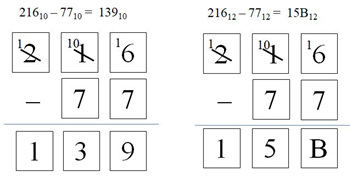In the example above, we aim to find the solution to 21612 – 7712. In the ones place, we cannot take 7 away from 6, so we have to “borrow” 12 from the twelves place. Hence,     1612 – 712 = B12. In the twelves place, we cannot take 7 away from the remaining zero. Thus, we “borrow” 144 from the hundred-forty-fours place, and hence 10012 – 7012 = 5012. We thus solve the problem: 21612 – 7712 = 15B12.

We can think of this as

(2×144) + (1×12) + (6×1) – (7×12) – (7×1) = (1×144) + (5×12) + (11×12).

This website provides a useful calculator for arithmetic in different bases:

https://www.rapidtables.com/calc/math/base-calculator.html

1. Solve the following problems, without converting to base-10:

368 + 148 = ?

43A12 + 11812 = ?

1110101002 – 1110012 = ?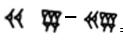= ?

2. Base-16 is used in computing, as base-2 digits can easily be converted to base-16. In base-16, the digits 0 to 9 represent the quantities from 0 to 9, while A, B, C, D, E, and F represent the quantities through 10 to 15 respectively. Convert the following numbers from base-2 to base-16:

10112

101100102

1011001011112

What do you notice? What is an advantage of using base-16 over base-2?

3. In Chinese, the characters for one (一), two (二), and three (三), consist of one, two, and three horizontal lines respectively. This could have been an example of a base-1 number system. A base-1 number system has only one digit, 1. Each place is worth one times as much as the place to the right of it, thus all places are worth 1. The value of the number is equal to the number of 1s in the number, for example

111111 = 5, and 11111111111 = 10

The following is a Chinese joke:

A man sends his child to learn Chinese. After the first day, he has learnt how to write “one”, “two”, and “three” in Chinese. Identifying the pattern, he tells his father that he is fluent in Chinese. His father thus asks him to write a letter to a family friend, Mr Wan (in Chinese, the character 万, a common surname, also means ten thousand). Five hours later the child is still writing. He exclaims: “Father, why must your friend be called Mr Wan? I have only written three thousand strokes!”

Identify two problems with a base-1 number system.

1. 368 + 148 = 528

43A12 + 11812 = 55612

1110101002 – 1110012 = 1100110112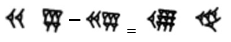2. 10112 = B16

101100102 = B216

1011001011112 = B2F16

The base-2 digits can be grouped into groups of four and converted into base-16 digits group by group. One advantage of base-16 over base-2 is that it requires less digits to write the same number.

3. As mentioned in the story, one problem is that many digits are needed to represent large numbers. To write “ten thousand” in base-1, ten thousand digits are needed, compared to just five digits in base-10.

Additionally, if all digits are worth 1, then it is impossible to represent any numbers less than 1 in base-1. Thus, there would be no decimals or fractions.

Author